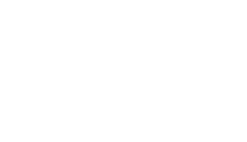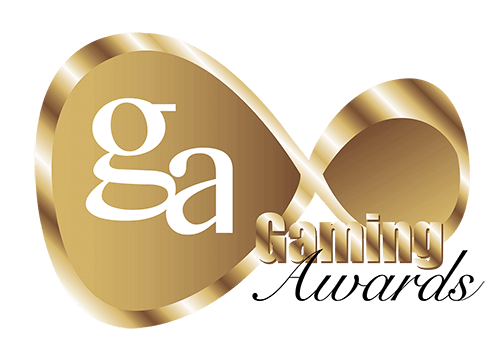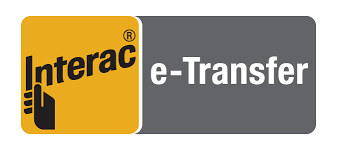# The Odds of Making a Straight in Poker

Font Size
A A A

The odds of flopping a Straight with a premium connector is 1.29% or 1 in 77.5

Definition of Straight

A Straight is formed with 5 cards all of consecutive rank.

Example – JT987

Aces can be both high and low for the sake of a Straight but must be either at the start or end of a run. I.e. QKA23 is not a legal Straight since the Ace falls in the middle of the run.

## Odds of Making a Straight on the Flop

Flopping a Straight is Hold’em is not overall that likely. Naturally, it depends on the type of starting hand we have.

Odds of flopping a Straight with any starting hand = 0.39%

Odds of flopping a Straight with a connector between 54 and JT = 1.29%

Odds of flopping a Straight with any connector = 1.04%

Odds of flopping a Straight with a one-gapper between 53 and QT = 0.96%

Odds of flopping a Straight with any one-gapper = 0.85%

Odds of flopping a Straight with a two-gapper between 52 and KT = 0.64%

Odds of flopping a Straight with any two-gapper = 0.61%

Odds of flopping a Straight with unconnected cards = 0%

Odds of flopping a Straight with a pocket pair = 0%

Why do we differentiate between 54-JT and other connectors? Simply because connectors between 54 and JT make the maximum number of Straights. A holding such as AK can only make the AKQJT Straight and is hence less likely to flop a Straight.

Odds of flopping a Straight with T9s = 1.29%

Odds of flopping a Straight with AKs = 0.32%

## Odds of Making a Straight Draw on the Flop

While making a Straight is unlikely, Straight draws are extremely playable holdings which often allow us to get past the flop. The odds of flopping different types of Straight draw may hence prove useful.

Odds of flopping any Straight draw with 54 to JT connectors = 26.2%

Odds of flopping an OESD with 54 to JT connectors = 9.6%

Odds of flopping a gutshot with 54 to JT connectors = 16.6%

Odds of flopping any Straight draw with 53 to QT one-gappers = 21.9%

Odds of flopping an OESD with 53 to QT one-gappers = 7.26%

Odds of flopping a gutshot with 53 to QT one-gappers = 14.6%

Odds of flopping any Straight draw with 63 to KT two-gappers = 17.9%

Odds of flopping any OESD with 63 to KT two-gappers = 4.47%

Odds of flopping any gutshot with 63 to KT two-gappers = 13.5%

Odds of flopping any Straight draw with a pocket pair = 4.12%

Odds of flopping any Straight draw with AK = 11.2%

Odds of flopping any Straight draw with T5o = 9.66%

It’s useful to know that we’ll actually hit a Straight draw a huge 26.2% of the time when holding a premium suited connector. Naturally, the chances of flopping a Straight draw decrease as our holdings become less connected.

The likelihood of unconnected hands picking up a Straight draw can vary. T5o might pick up a Straight draw 9.66% of the time, but A9 only picks up a Straight draw 6.53% of the time. Middling cards are better at flopping Straight draws than high or low cards.

## Odds of Making a Straight on the Later Streets

The probability of making a Straight on the later streets depends on the type of draw we flop. Open-ended Straight draws (OESD) have 8 outs, while gutshots have 4 outs.

Odds of hitting from flop to turn

Odds of hitting an open-ended Straight draw from flop to turn = 8/47 = 0.1702 or about 17%

Odds of hitting a gutshot from flop to turn = 4/47 = 0.0851 or about 8.5%

Odds of hitting from turn to river

Odds of hitting an open-ended Straight draw from turn to river = 8/46 = 0.1739 or about 17.4%

Odds of hitting a gutshot from turn to river = 4/46 = 0.087 or about 8.7%

Odds of hitting from flop to river

Here we will use the simple trick of calculating the probability of not hitting and deducting it from 100%.

Probability of not hitting an OESD on the turn = 39/47

Probability of not hitting an OESD on the river = 38/46

Probability of not hitting a gutshot on the turn or river = 39/47 * 38/46 = 0.6855 or 68.5%

Probability of not hitting a gutshot on the turn = 43/47

Probability of not hitting a gutshot on the river = 42/46

Probability of not hitting a gutshot on the turn or river = 43/47 * 42/46 = 0.8353 or 83.5%

Therefore -

The probability of hitting a gutshot on the turn or river = (100 – 83.5) roughly 26.5%

The probability of hitting an OESD on the turn or river = (100 – 68.5) roughly 31.5%

## Implied Odds Analysis of a Straight

Straights are powerful hands in Hold’em and are nearly always good enough to invest our 100bb stack on when hitting. Hence, they have exceptional implied odds.

However, it’s important to remember that not all Straights are equal in the implied odds department. There are several important factors to be kept in mind.

Nutted-ness – A nut Straight is always more valuable than a non-nut Straight. For example, on a 789 texture, the implied odds value of a Straight will be ranked in the following order, from highest to lowest.

Texture 789

Hand 1 - JT -- Best implied odds. This hand will beat all other Straights on the texture.

Hand 2 - T6 – In the middle.

Hand 3 - 65 – Worst implied odds. This hand may end up dominated by better Straights.

2-card or 1-card – Straights vary heavily in strength based on whether they are formulated with one or both of our hole cards. Straights formed with only one of our hole cards implies that there must be a “four to Straight” texture on the board such as 5678x, or TJKAx.

In such circumstances, our Straight is more visible, or “transparent” since only one card is needed. Therefore, our opponent may easily give us more credit for making the Straight (making it harder to get paid off).

To make a Straight on a texture such as QT8xx requires us to hold two cards of precise rank, so our opponent may be less inclined to give us credit (and hence more likely to pay us off).

For the most part, Straights in Hold’em are monsters, and we should not be looking to fold them at 100bb effective stacks.

However, certain weaker Straights might not be worth losing our stack over - specifically, the non-nut one-card Straight.

Example: Board 789T42

Our Hand: 63

Yes, we have a Straight here, but our opponents just need a single Jack in the hole to make a higher Straight.

In such circumstances, we are not necessarily committed with our Straight assuming 100bb effective stacks (although it naturally depends somewhat on the action).

 Method (Straight) Probability (%) Flopping a Straight with any starting hand 0.39 Flopping a Straight with a connector from 54 to JT 1.29 Flopping a Straight with any connector 1.04 Flopping a Straight with a one-gapper from 53 to QT 0.96 Flopping a Straight with any one-gapper 0.85 Flopping a Straight with any  two-gapper from 52 to KT 0.64 Flopping a Straight with any two-gapper 0.61 Flopping a Straight with unconnected cards 0 Flopping a Straight with a pocket pair 0 Floppping a Straight with T9s 1.29 Flopping a Straight with Aks 0.32 Flopping a Straight draw with connectors from 54 to JT 26.2 Flopping an OESD with connectors from 54 to JT 9.6 Flopping a Gutshot with connectors from 54 to JT 16.6 Flopping a Straight draw with one-gappers from 53 to QT 21.9 Flopping an OESD with one-gappers from 53 to QT 7.26 Flopping a Gutshot with one-gappers from 53 to QT 14.6 Flopping a Straight draw with two-gappers from 63 to KT 17.9 Flopping an OESD with two-gappers from 63 to KT 4.47 Flopping a Gutshots with two-gappers from 63 to KT 13.5 Flopping a Straight draw with a pocket pair 4.12 Flopping a Straight draw with AK 11.2 Flopping a Straight draw with T5o 9.66 Hitting an OESD from flop to turn 17 Hitting an OESD from turn to river 17.4 Hitting an OESD from flop to river 31.5 Hitting a Gutshot from flop to turn 8.5 Hitting a Gutshot from turn to river 8.7 Hitting a Gutshot from flop to river 26.5
Awards